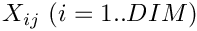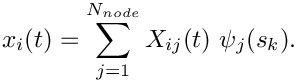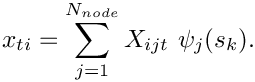oomph::PMLMapping Class Referenceabstract

Class to hold the mapping function (gamma) for the Pml which defines how the coordinates are transformed in the Pml. This class holds the one dimensional or uniaxial case which is the most common. More...

#include <pml_mapping_functions.h>Inheritance diagram for oomph::PMLMapping:

## Public Member Functions

PMLMapping ()
Default constructor (empty) More...

virtual std::complex< double > gamma (const double &nu_i, const double &pml_width_i, const double &wavenumber_squared, const double &alpha_shift=0.0)=0
Pure virtual to return Pml mapping gamma, where gamma is theas function ofwherewhere h is the vector from the origin to the start of the Pml. More...

## Detailed Description

Class to hold the mapping function (gamma) for the Pml which defines how the coordinates are transformed in the Pml. This class holds the one dimensional or uniaxial case which is the most common.

Definition at line 45 of file pml_mapping_functions.h.

## ◆ PMLMapping()

 oomph::PMLMapping::PMLMapping ( )
inline

Default constructor (empty)

Definition at line 49 of file pml_mapping_functions.h.

## ◆ gamma()

 virtual std::complex< double > oomph::PMLMapping::gamma ( const double & nu_i, const double & pml_width_i, const double & wavenumber_squared, const double & alpha_shift = 0.0 )
pure virtual

Pure virtual to return Pml mapping gamma, where gamma is theas function ofwherewhere h is the vector from the origin to the start of the Pml.

Implemented in oomph::BermudezPMLMapping, and oomph::ContinuousBermudezPMLMapping.

The documentation for this class was generated from the following file: